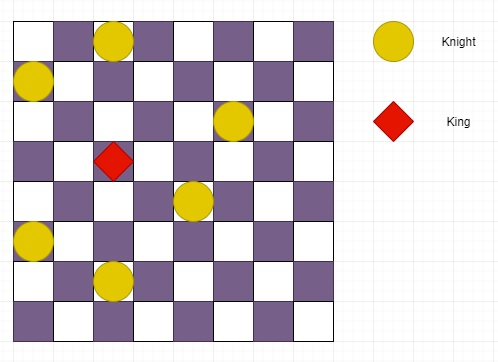# Check if a king can move a valid move or not when N nights are there in a modified chessboard in Python

Suppose we have one infinite chessboard with the same rules as that of chess and if there are N knights coordinates on the infinite chessboard and the king’s coordinate, we have to check whether the King is checkmate or not. The coordinate of infinite board is bounded by large value like (-10^9 <= x, y <= 10^9).

So, if the input is like knights position = [[2,1],[1,3],[3,6],[5,5],[6,1],[7,3]] and king position: [4,3],then the output will be True, as king has no move, so it is check mate.

To solve this, we will follow these steps −

• my_dict := a new map
• for i in range 0 to n, do
• x := a[i, 0]
• y := a[i, 1]
• my_dict[x, y] := 1
• my_dict[x - 2, y + 1] := 1
• my_dict[x - 2, y - 1] := 1
• my_dict[x + 1, y + 2] := 1
• my_dict[x + 1, y - 2] := 1
• my_dict[x - 1, y + 2] := 1
• my_dict[x + 2, y + 1] := 1
• my_dict[x + 2, y - 1] := 1
• my_dict[x - 1, y - 2] := 1
• for i in range -1 to 1, do
• for j in range -1 to 1, do
• nx := king_pos + i
• ny := king_pos + j
• if i is not same as 0 and j is not same as 0, then
• if my_dict[(nx, ny)] is zero, then
• return False
• return True

## Example

Let us see the following implementation to get better understanding −

Live Demo

def is_checkmate(a, n, king_pos):
my_dict = {}
for i in range(0, n):
x = a[i]
y = a[i]
my_dict[(x, y)] = 1
my_dict[(x - 2, y + 1)] = 1
my_dict[(x - 2, y - 1)] = 1
my_dict[(x + 1, y + 2)] = 1
my_dict[(x + 1, y - 2)] = 1
my_dict[(x - 1, y + 2)] = 1
my_dict[(x + 2, y + 1)] = 1
my_dict[(x + 2, y - 1)] = 1
my_dict[(x - 1, y - 2)] = 1
for i in range(-1, 2):
for j in range(-1, 2):
nx = king_pos + i
ny = king_pos + j
if i != 0 and j != 0:
if not my_dict[(nx, ny)]:
return False
return True
a = [[2,1],[1,3],[3,6],[5,5],[6,1],[7,3]]
n = len(a)
pos = [4, 3]
print (is_checkmate(a, n, pos))

## Input

[[2,1],[1,3],[3,6],[5,5],[6,1],[7,3]], 6, [4, 3]

## Output

True